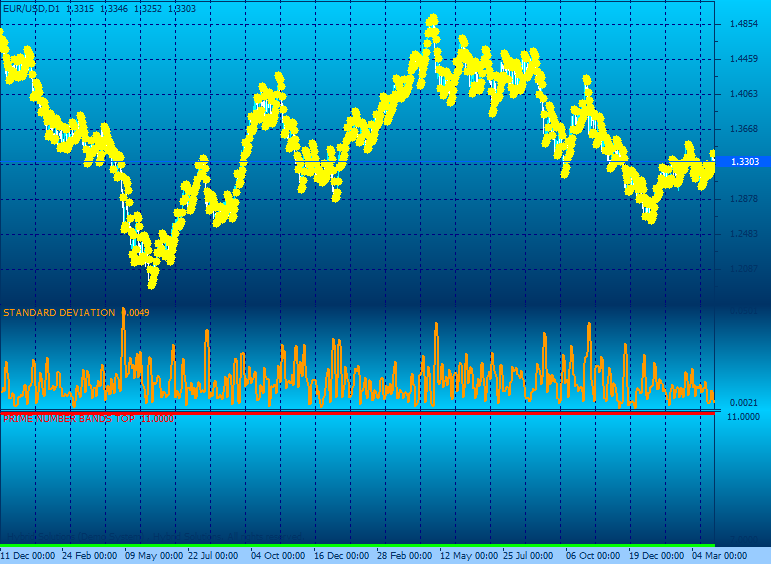## SAR Prime Numbers Template

Image\$Free
Hits
7426
RatingOn
29 May 2012
Attachment (Desktop)
Compatibility

## Contains

• Parabolic Sar: idea of an indicator is to buy into up trends when the indicator is below price, and sell into down trends when the indicator is above price;
• Standard Deviation: measures how widely values (closing prices for instance) are dispersed from the average;
• Prime Number Bands: identifies the nearest prime number for the high and low and plots the two series as bands.

## Parameters

• Parabolic SAR: Step (float), Maximum (float);
• Standard Deviation: Period (integer); Method (Simple, Exponential, Time Series, Variable, VIDYA, Weighted), Stand Dev (integer);
• Prime Number Bands.

## Image